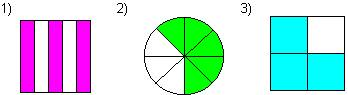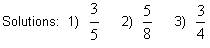# Fractions Math Review - Introduction to Fractions

math tutorials > Introduction to Fractions

## What are fractions?

Fractions are part of a whole.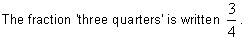The bottom number is called the denominator.
The top number is called the numerator.

Fractions can be illustrated with diagrams.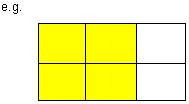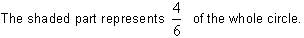The circle has been divided into 6 equal parts, 4 of which have been shaded.

## Fraction problems for you to try.

Express the shaded area as a fraction of the whole.